# Addition of 2-digit numbers with Regrouping | Maths Grade2

### Addition of 2-digit numbers with Regrouping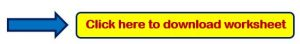Example 1:- Add the following?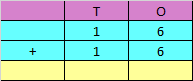Explanation:

Step 1: Adding the digits at one’s place,

6 + 6 = 12

Since the number is more than 9, we need to carry over 1 to the ten’s place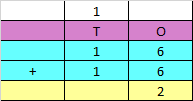Step 2: Adding the digits at ten’s place and the carry of 1,

1 + 1 + 1 = 3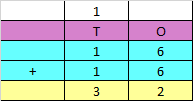Hence, the sum of the given numbers is 32

Example 2:- Add the following?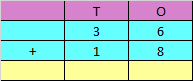Explanation:

Step 1: Adding the digits at one’s place,

6 + 8 = 14

Since the number is more than 9, we need to carry over 1 to the ten’s place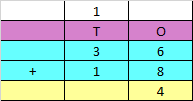Step 2: Adding the digits at tens place and the carry of 1,

3 + 1 + 1 = 5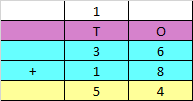Hence, the sum of the given numbers is 54

Example 3:- Add the following?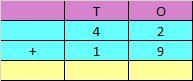Explanation:

Step 1: Adding the digits at one’s place,

2 + 9 = 11

Since the number is more than 9, we need to carry over 1 to the ten’s place.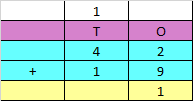Step 2: Adding the digits at tens place and the carry of 1,

4 + 1 + 1 = 6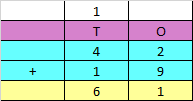Hence, the sum of the given numbers is 61